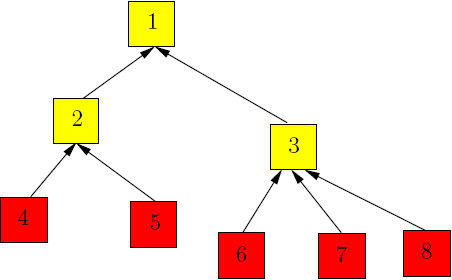# Lecture #3: PageRank Algorithm - The Mathematics of Google Search

We live in a computer era. Internet is part of our everyday lives and information is only a click away. Just open your favorite search engine, like Google, AltaVista, Yahoo, type in the key words, and the search engine will display the pages relevant for your search. But how does a search engine really work?

At first glance, it seems reasonable to imagine that what a search engine does is to keep an index of all web pages, and when a user types in a query search, the engine browses through its index and counts the occurrences of the key words in each web file. The winners are the pages with the highest number of occurrences of the key words. These get displayed back to the user.

This used to be the correct picture in the early 90s, when the first search engines used text based ranking systems to decide which pages are most relevant to a given query. There where however a number of problems with this approach. A search about a common term such as "Internet" was problematic. The first page displayed by one of the early search engines was written in Chinese, with repeated occurrences of the word "Internet" and containing no other information about the Internet. Moreover, suppose we wanted to find some information about Cornell. We type in the word "Cornell" and expect that "www.cornell.edu" would be the most relevant site to our query. However there may be millions of pages on the web using the world Cornell, and www.cornell.edu may not be the one that uses it most often. Suppose we decided to write a web site that contains the word "Cornell" a billion times and nothing else. Would it then make sense for our web site to be the first one displayed by a search engine? The answer is obviously no. However, if all a search engine does is to count occurrences of the words given in the query, this is exactly what might happen.

The usefulness of a search engine depends on the relevance of the result set it gives back. There may of course be millions of web pages that include a particular word or phrase; however some of them will be more relevant, popular, or authoritative than others. A user does not have the ability or patience to scan through all pages that contain the given query words. One expects the relevant pages to be displayed within the top 20-30 pages returned by the search engine.

Modern search engines employ methods of ranking the results to provide the "best" results first that are more elaborate than just plain text ranking. One of the most known and influential algorithms for computing the relevance of web pages is the Page Rank algorithm used by the Google search engine. It was invented by Larry Page and Sergey Brin while they were graduate students at Stanford, and it became a Google trademark in 1998. The idea that Page Rank brought up was that, the importance of any web page can be judged by looking at the pages that link to it. If we create a web page i and include a hyperlink to the web page j, this means that we consider j important and relevant for our topic. If there are a lot of pages that link to j, this means that the common belief is that page j is important. If on the other hand, j has only one backlink, but that comes from an authoritative site k, (like www.google.com, www.cnn.com, www.cornell.edu) we say that k transfers its authority to j; in other words, k asserts that j is important. Whether we talk about popularity or authority, we can iteratively assign a rank to each web page, based on the ranks of the pages that point to it.

To this aim, we begin by picturing the Web net as a directed graph, with nodes represented by web pages and edges represented by the links between them.

Suppose for instance, that we have a small Internet consisting of just 4 web sites www.page1.com, www.page2.com, www.page3.com, www.page4.com, referencing each other in the manner suggested by the picture:We "translate" the picture into a directed graph with 4 nodes, one for each web site. When web site i references j, we add a directed edge between node i and node j in the graph. For the purpose of computing their page rank, we ignore any navigational links such as back, next buttons, as we only care about the connections between different web sites. For instance, Page1 links to all of the other pages, so node 1 in the graph will have outgoing edges to all of the other nodes. Page3 has only one link, to Page 1, therefore node 3 will have one outgoing edge to node 1. After analyzing each web page, we get the following graph:In our model, each page should transfer evenly its importance to the pages that it links to. Node 1 has 3 outgoing edges, so it will pass onof its importance to each of the other 3 nodes. Node 3 has only one outgoing edge, so it will pass on all of its importance to node 1. In general, if a node has k outgoing edges, it will pass onof its importance to each of the nodes that it links to. Let us better visualize the process by assigning weights to each edge.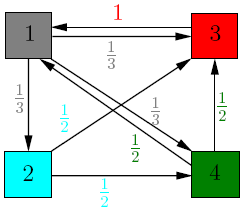Let us denote by A the transition matrix of the graph, A =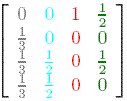.

Dynamical systems point of view:

Suppose that initially the importance is uniformly distributed among the 4 nodes, each getting ¼. Denote by v the initial rank vector, having all entries equal to ¼. Each incoming link increases the importance of a web page, so at step 1, we update the rank of each page by adding to the current value the importance of the incoming links. This is the same as multiplying the matrix A with v . At step 1, the new importance vector is v1 = Av. We can iterate the process, thus at step 2, the updated importance vector is v2 = A(Av) = A2v. Numeric computations give: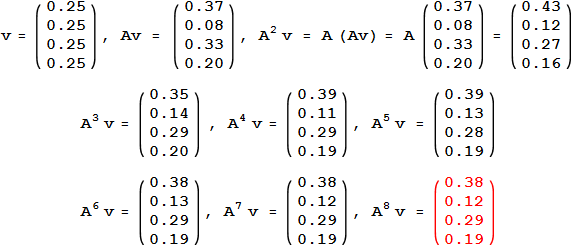We notice that the sequences of iterates v, Av, ..., Akv tends to the equilibrium value v* =. We call this the PageRank vector of our web graph.

Linear algebra point of view:

Let us denote by x1, x2, x3, and x4 the importance of the four pages. Analyzing the situation at each node we get the system: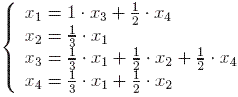This is equivalent to asking for the solutions of the equations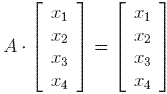. From Example 6 in Lecture 1 we know that the eigenvectors corresponding to the eigenvalue 1 are of the form. Since PageRank should reflect only the relative importance of the nodes, and since the eigenvectors are just scalar multiples of each other, we can choose any of them to be our PageRank vector. Choose v* to be the unique eigenvector with the sum of all entries equal to 1. (We will sometimes refer to it as the probabilistic eigenvector corresponding to the eigenvalue 1). The eigenvectoris our PageRank vector.

Probabilistic point of view:

Since the importance of a web page is measured by its popularity (how many incoming links it has), we can view the importance of page i as the probability that a random surfer on the Internet that opens a browser to any page and starts following hyperlinks, visits the page i. We can interpret the weights we assigned to the edges of the graph in a probabilistic way: A random surfer that is currently viewing web page 2, has ½ probability to go to page 3, and ½ probability to go to page 4. We can model the process as a random walk on graphs. Each page has equal probability ¼ to be chosen as a starting point. So, the initial probability distribution is given by the column vector [¼ ¼ ¼ ¼]t. The probability that page i will be visited after one step is equal to Ax, and so on. The probability that page i will be visited after k steps is equal to Akx. The sequence Ax, A2x, A3x, ..., Akx, ... converges in this case to a unique probabilistic vector v*. In this context v* is called the stationary distribution and it will be our Page Rank vector. Moreover, the ith entry in the vector v* is simply the probability that at each moment a random surfer visits page i. The computations are identical to the ones we did in the dynamical systems interpretation, only the meaning we attribute to each step being slightly different.

The Page Rank vector v* we have computed by different methods, indicates that page 1 is the most relevant page. This might seem surprising since page 1 has 2 backlinks, while page 3 has 3 backlinks. If we take a look at the graph, we see that node 3 has only one outgoing edge to node 1, so it transfers all its importance to node 1. Equivalently, once a web surfer that only follows hyperlinks visits page 3, he can only go to page 1. Notice also how the rank of each page is not trivially just the weighted sum of the edges that enter the node. Intuitively, at step 1, one node receives an importance vote from its direct neighbors, at step 2 from the neighbors of its neighbors, and so on.

Changing the web graph might lead to certain problems.

Nodes with no outgoing edges (dangling nodes)We iteratively compute the rank of the 3 pages: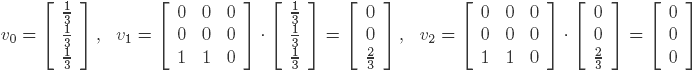.

So in this case the rank of every page is 0. This is counterintuitive, as page 3 has 2 incoming links, so it must have some importance!

An easy fix for this problem would be to replace the column corresponding to the dangling node 3 with a column vector with all entries 1/3. In this way, the importance of node 3 would be equally redistributed among the other nodes of the graph, instead of being lost.

Disconnected componentsA random surfer that starts in the first connected component has no way of getting to web page 5 since the nodes 1 and 2 have no links to node 5 that he can follow. Linear algebra fails to help as well. The transition matrix for this graph is. Notice that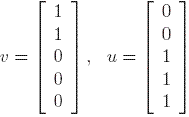are both eigenvectors corresponding to the eigenvalue 1, and they are not just trivially one the a scalar multiple of the other. So, both in theory and in practice, the notation of ranking pages from the first connected component relative to the ones from the second connected component is ambiguous.

The web is very heterogeneous by its nature, and certainly huge, so we do not expect its graph to be connected. Likewise, there will be pages that are plain descriptive and contain no outgoing links. What is to be done in this case? We need a non ambiguous meaning of the rank of a page, for any directed Web graph with n nodes.

The solution of Page and Brin:

In order to overcome these problems, fix a positive constant p between 0 and 1, which we call the damping factor (a typical value for p is 0.15). Define the Page Rank matrix (also known as the Google matrix) of the graph by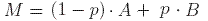where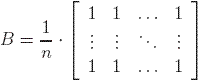.

Problem 1. Prove that M remains a column stochastic matrix. Prove that M has only positive entries.

The matrix M models the random surfer model as follows: most of the time, a surfer will follow links from a page: from a page i the surfer will follow the outgoing links and move on to one of the neighbors of i. A smaller, but positive percentage of the time, the surfer will dump the current page and choose arbitrarily a different page from the web and "teleport" there. The damping factor p reflects the probability that the surfer quits the current page and "teleports" to a new one. Since he/she can teleport to any web page, each page hasprobability to be chosen. This justifies the structure of the matrix B.

Problem 2. Redo the computations for the Page Rank with the transition matrix A replaced with the matrix M, for the graphs representing the Dangling nodes, respectively Disconnected components. Do the problems mentioned in there still occur?

Intuitively, the matrix M "connects" the graph and gets rid of the dangling nodes. A node with no outgoing edges has nowprobability to move to any other node. Rigorously, for the matrix M, the following theorems apply:

Perron-Frobenius Theorem: If M is a positive, column stochastic matrix, then:
1. 1 is an eigenvalue of multiplicity one.
2. 1 is the largest eigenvalue: all the other eigenvalues have absolute value smaller than 1.
3. the eigenvectors corresponding to the eigenvalue 1 have either only positive entries or only negative entries. In particular, for the eigenvalue 1 there exists a unique eigenvector with the sum of its entries equal to 1.
Power Method Convergence Theorem: Let M be a positive, column stochastic n × n matrix. Denote by v* its probabilistic eigenvector corresponding to the eigenvalue 1. Let z be the column vector with all entries equal to. Then the sequence z, Mz, ..., Mkz converges to the vector v*.

In view of everything discussed above, we conclude that:

Fact: The PageRank vector for a web graph with transition matrix A, and damping factor p, is the unique probabilistic eigenvector of the matrix M, corresponding to the eigenvalue 1.

From the mathematical point of view, once we have M, computing the eigenvectors corresponding to the eigenvalue 1 is, at least in theory, a straightforward task. As in Lecture 1, just solve the system Ax = x! But when the matrix M has size 30 billion (as it does for the real Web graph), even mathematical software such as Matlab or Mathematica are clearly overwhelmed.

An alternative way of computing the probabilistic eigenvector corresponding to the eigenvalue 1 is given by the Power Method. The theorem guarantees that the method works for positive, column stochastic matrices. We reasoned that the iteration process corresponds to the way importance distributes over the net following the link structure (Recall the random surfer model). Computationally speaking, it is much more easier, starting from the vector with all entries 1, to multiply x, Mx,..., Mnx until convergence then it is to compute the eigenvectors of M. In fact, in this case, one needs only compute the first couple of iterates in order to get a good approximation of the PageRank vector. For a random matrix, the power method is in general known to be slow to converge. What makes it work fast in this case however is the fact that the web graph is sparse. This means that a node i has a small number of outgoing links (a couple of hundred at best, which is extremely small corresponding to the 30 billion nodes it could theoretically link to). Hence the transition matrix A has a lot of entries equal to 0.

We end the lecture by proposing the following problems:

Problem 3. Compute the PageRank vector of the following graph, considering the damping constant p to be successively p = 0, p = 0.15, p = 0.5, and respectively p = 1.Problem 4. Compute the PageRank vector of the directed tree depicted below, considering that the damping constant p = 0.15. Interpret your results in terms of the relationship between the number of incoming links that each node has and its rank.